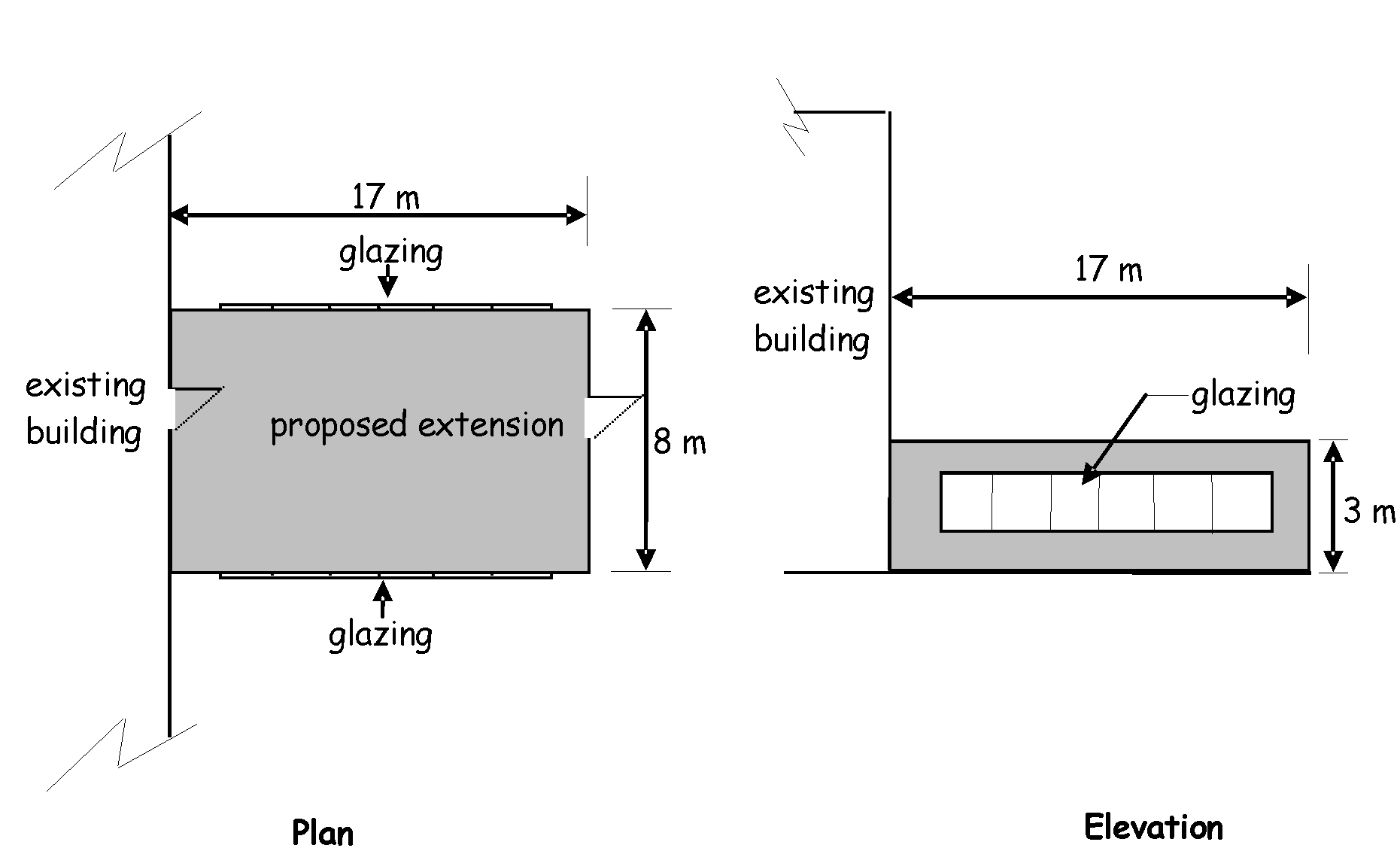# Building standards technical handbook 2020: non-domestic

The building standards technical handbooks provide guidance on achieving the standards set in the Building (Scotland) Regulations 2004. This handbook applies to a building warrant submitted on or after 1 March 2021 and to building work which does not require a warrant commenced from that date.

## Annex 6.B Compensatory approach - heat loss example

### 6.B.0 Introduction

This annex gives an example of the compensatory approach which can be used in the design of conversions, extensions and alterations. This is likely to be of use where there is a need to specify one or more constructions with a U-value higher than the recommended maximum area-weighted average U-values given in column (a) of the table to clause 6.2.11.

The example given in this instance is for an extension. However the same principles are relevant to a conversion or to substantial alterations.

Note that this method cannot be used in conversions, if recommended U-values are only being met as far as is reasonably practicable.

Separate work under the same building warrant - a single compensatory approach calculation can be carried out to cover separate areas of work to an existing building provided the same assessment criteria (maximum U-values, etc) are applicable to each area of work.

### 6.B.1 Example - extension to an existing building

It is proposed to form a 3m high extension onto an existing single storey office building. The extra floor area created will be 136m2. A plan of the proposed layout is shown in the figure below. In this example the principle compensatory measure is the omission of roof-lights, as the designer considers that it is easier to provide solar shading to slightly larger windows. The existing building and extension will be heated to a similar degree so there is no heat loss considered between these 2 areas.

Procedure:

• The internal exposed surface areas of each of the elements of the proposed building insulation envelope that have different area weighted U-values are calculated.

• The heat loss for the proposed extension is calculated using proposed U-values for building elements, which may be higher or lower than those recommended in column (a) of the table to clause 6.2.11. The percentage area of windows and doors area as proposed may be greater than the maximum percentage area of the exposed wall area noted in clause 6.2.11.

• The heat loss for a ‘notional’ extension (i.e. a building of the same size and shape as the proposed but with its area window and doors at the maximum percentage area noted under clause 6.2.11) is calculated using the maximum area-weighted average U-values in the table to clause 6.2.11.

• Finally, the heat loss calculated for the proposed building should be not more than that for the 'notional' one.

Figure 6.1. Extension example### 6.B.2 Proposed extension

From the information in 6.B.1 the rate of heat loss from the proposed extension is then calculated as follows:

Table 6.9. Proposed extension heat loss calculation

Exposed Element Exposed surface area (m2) U-value (W/m2 K) Rate of heat loss (W/K)
External wall 126-63 = 63 x 0.22 = 13.86
Roof 136.0 x 0.16 = 21.76
Floor 136.0 x 0.22 = 29.92
External Door 3.0 x 1.8 = 5.4
Window 2 of (15x2m) = 60.0 x 1.8 = 108
Roof-light Not applicable
Total rate of heat loss = 178.94

### 6.B.3 'Notional extension'

The rate of heat loss from the notional extension is then calculated as follows:

Table 6.10. Notional extension heat loss calculation

Exposed Element Exposed surface area (m2) U-value (W/m2 K) Rate of heat loss (W/K)
External wall 126 - 50.4 = 75.6 x 0.25 = 18.90
Roof 136 - 27.2 =108.8 x 0.15 = 16.32
Floor 136 x 0.20 = 27.20
Window + Ext. Door 50.4 (40%) x 1.6 = 80.64
Roof-light 27.2 (20%) x 1.6 = 43.52
Total rate of heat loss = 186.58

### 6.B.4 The comparison

The rate of heat loss from the proposed extension (178.94) is less than that from the ‘notional extension’ (186.58). Proposals will comply.